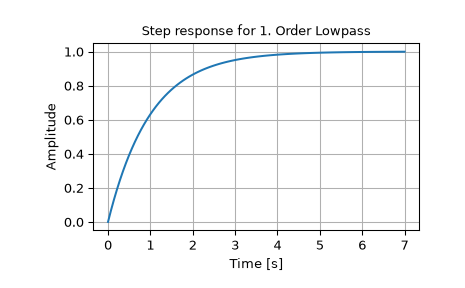# scipy.signal.step¶

scipy.signal.step(system, X0=None, T=None, N=None)[source]

Step response of continuous-time system.

Parameters
systeman instance of the LTI class or a tuple of array_like

describing the system. The following gives the number of elements in the tuple and the interpretation:

• 1 (instance of `lti`)

• 2 (num, den)

• 3 (zeros, poles, gain)

• 4 (A, B, C, D)

X0array_like, optional

Initial state-vector (default is zero).

Tarray_like, optional

Time points (computed if not given).

Nint, optional

Number of time points to compute if T is not given.

Returns
T1D ndarray

Output time points.

yout1D ndarray

Step response of system.

Notes

If (num, den) is passed in for `system`, coefficients for both the numerator and denominator should be specified in descending exponent order (e.g. `s^2 + 3s + 5` would be represented as `[1, 3, 5]`).

Examples

```>>> from scipy import signal
>>> import matplotlib.pyplot as plt
>>> lti = signal.lti([1.0], [1.0, 1.0])
>>> t, y = signal.step(lti)
>>> plt.plot(t, y)
>>> plt.xlabel('Time [s]')
>>> plt.ylabel('Amplitude')
>>> plt.title('Step response for 1. Order Lowpass')
>>> plt.grid()
```